Share

Lakhmir Singh solutions for Class 9 Science chapter 2 - Force and Laws of Motion

Science for Class 9 Part 1 Physics

Lakhmir Singh Science Class 9 Part 1 Physics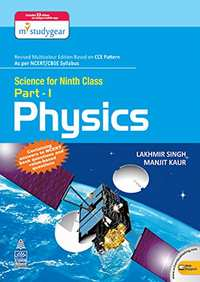Chapter 2: Force and Laws of Motion

Chapter 2: Force and Laws of Motion Exercise Very Short Answers, Short Answers, Long Answers, MCQ, Hots Questions solutions [Pages 55 - 57]

Very Short Answers | Q 1 | Page 55

What name is given to the product of mass and velocity of a body ?

Very Short Answers | Q 2 | Page 55

Name the physical quantity which is considered to be a measure of the quantity of motion of a body.

Very Short Answers | Q 3 | Page 55

What is the SI unit of momentum ?

Very Short Answers | Q 4 | Page 55

state whether momentum is scalar or vector.

Very Short Answers | Q 5 | Page 55

What is the total momentum of the bullet and the gun before firing ?

Very Short Answers | Q 6 | Page 55

Name the physical quantity whose unit is kg.m/s.

Very Short Answers | Q 7 | Page 55

What will be the momentum of a body of mass ‘m’ which is moving with a velocity V ?

Very Short Answers | Q 8 | Page 55

What is the usual name of the forces which cannot produce motion in a body but only change its shape ?

Very Short Answers | Q 9 | Page 55

Name the unbalanced force which slows down a moving bicycle when we stop pedalling it.

Very Short Answers | Q 10 | Page 55

State whether the following statement is true or false :
Unbalanced forces acting on a body change its shape.

Very Short Answers | Q 11 | Page 55

When a ball is dropped from a height, its speed increases gradually. Name the force which causes this change in speed.

Very Short Answers | Q 12 | Page 55

Name the property of bodies (or objects) to resist a change in their state of rest or of motion.

Very Short Answers | Q 13 | Page 55

What is the other name of Newton’s first law of motion ?

Very Short Answers | Q 14 | Page 55

The mass of object A is 6 kg whereas that of another object B is 34 kg. Which of the two objects, A or B, has more inertia ?

Very Short Answers | Q 15 | Page 55

Name the scientist who gave the laws of motion.

Very Short Answers | Q 16 | Page 55

State whether force is a scalar or a vector quantity?

Very Short Answers | Q 17 | Page 55

With which physical quantity should the speed of a running bull be multiplied so as to obtain its momentum ?

Very Short Answers | Q 18.1 | Page 55

Fill in the following blank with suitable word :
(a) ………………is a measure of the inertia of a body.

Very Short Answers | Q 18.2 | Page 55

Fill in the following blank with suitable word:

When a running car stops suddenly, the passengers are jerked …………………..

Very Short Answers | Q 18.3 | Page 55

Fill in the following blank with suitable word :

When a stationary car starts suddenly, the passengers are jerked …………………..

Very Short Answers | Q 18.4 | Page 55

Fill in the following blank with suitable word :

Newton’s first law of motion is also called Galileo’s law of ………………………

Very Short Answers | Q 18.5 | Page 55

Fill in the following blanks with suitable word :

If there were no unbalanced force of……………….. and no…………………. resistance, a moving bicycle would go on moving for ever.

Short Answers | Q 19 | Page 55

Explain why, it is easier to stop a tennis ball than a cricket ball moving with the same speed.

Short Answers | Q 20 | Page 55

Explain the meaning of the following equation : .
p = m x v
where symbols have their usual meanings.

Short Answers | Q 21 | Page 55

Explain how, a karate player can break a pile of tiles with a single blow of his hand.

Short Answers | Q 22 | Page 55

Calculate the momentum of a toy car of mass 200 g moving with a speed of 5 m/s.

Short Answers | Q 23 | Page 55

What is the change in momentum of a car weighing 1500 kg when its speed increases from 36 km/h to 72 km/h uniformly ?

Short Answers | Q 24 | Page 55

A body of mass 25 kg has a momentum of 125 kg.m/s. Calculate the velocity of the body.

Short Answers | Q 25 | Page 55

Calculate the momentum of the following :
(a) an elephant of mass 2000 kg moving at 5 m/s
(b) a bullet of mass 0.02 kg moving at 400 m/s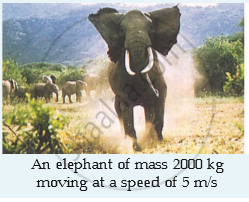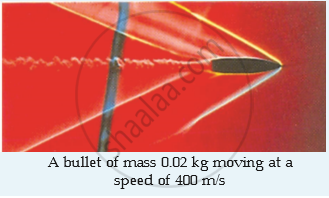Short Answers | Q 26 | Page 56

Which of the two, balanced forces or unbalanced forces, can change the shape of an object ? Give an example to illustrate your answer.

Short Answers | Q 27 | Page 56

Describe the term ‘inertia’ with respect to motion.

Short Answers | Q 28 | Page 56

State Newton’s first law of motion. Give two examples to illustrate Newton’s first law of motion.

Short Answers | Q 29 | Page 56

On what factor does the inertia of a body depend ? Which has more inertia, a cricket ball or a rubber ball of the same size ?

Short Answers | Q 30 | Page 56

Why do the passengers in a bus tend to fall backward when it starts suddenly ?

Short Answers | Q 31 | Page 56

Explain why, a person travelling in a bus falls forward when the bus stops suddenly.

Short Answers | Q 32 | Page 56

Give reason for the following :
When a hanging carpet is beaten with a stick, the dust particles start coming out of it.

Short Answers | Q 33 | Page 56

When a tree is shaken, its fruits and leaves fall down . Why ?

Short Answers | Q 34 | Page 56

Explain why, it is dangerous to jump out of a moving bus.

Short Answers | Q 35 | Page 56

What is the momentum in kg.m/s of a 10 kg car travelling at (a) 5 m/s (b) 20 cm/s, and (c) 36 km/h ?

Long Answers | Q 36.1 | Page 56

Define momentum of a body. On what factors does the momentum of a body depend ?

Long Answers | Q 36.2 | Page 56

Calculate the change in momentum of a body weighing 5 kg when its velocity decreases from 20 m/s to 0.20 m/s.

Long Answers | Q 37.1 | Page 56

Define the term ‘force’.

Long Answers | Q 37.2 | Page 56

State the various effects of force.

Long Answers | Q 38.1 | Page 56

Give one example  where  a force moves a stationary body.

Long Answers | Q 38.2 | Page 56

Give one example where a force stops a moving body .

Long Answers | Q 38.3 | Page 56

Give one example where a force changes the speed of a moving body.

Long Answers | Q 38.4 | Page 56

Give one example  where a force changes the direction of a moving body.

Long Answers | Q 38.5 | Page 56

Give one example where a force changes the shape (and size) of a body.

Long Answers | Q 39.1 | Page 56

What do you understand by the terms “balanced forces” and “unbalanced forces” ? Explain with examples.

Long Answers | Q 39.2 | Page 56

What type of forces – balanced or unbalanced – act on a rubber ball when we press it between our hands ? What effect is produced in the ball ?

Long Answers | Q 40.1 | Page 56

What happens to the passengers travelling in a bus when the bus takes a sharp turn ? Give reasons for your answer.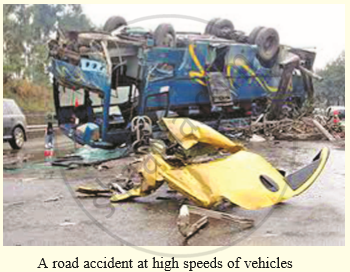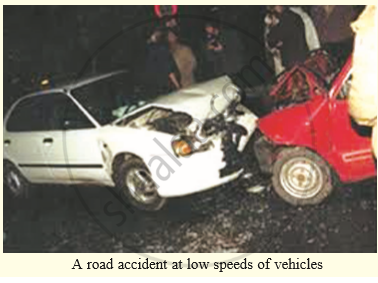Long Answers | Q 40.2 | Page 56

Why are road accidents at high speeds very much worse than road accidents at low speeds ?MCQ | Q 41 | Page 56

When a toothpaste tube is squeezed, its shape changes. The force responsible for this is an example of :

• balanced forces

• centripetal forces

• unbalanced forces

• centrifugal forces

MCQ | Q 42 | Page 57

The inertia of an object tends to cause an object :

• to increase its speed

• to decrease its speed

• to resist a change in its state of motion

• to decelerate due to friction

MCQ | Q 43 | Page 57

When we talk of a force acting on a body, it usually means :

• electrical force

• balanced force

• unbalanced force

• nuclear force

MCQ | Q 44 | Page 57

A passenger in a moving train tosses a coin which falls behind him. This shows that the motion of train is :

• accelerated

• uniform

• retarded

• along circular track

MCQ | Q 45 | Page 57

'When a hanging carpet is beaten with a stick, the dust particles start coming out of it'. This phenomenon can be best explained by making use of :

• Newton's third law of motion

• Newton's law of gravitation

• Newton's first law of motion

• Newton's second law of motion

MCQ | Q 46 | Page 57

A water tanker filled up to two-thirds of its tank with water is running with a uniform speed. When the brakes are suddenly applied, the water in its tank would :

• move backward

• move forward

• rise upwards

• remain unaffected

MCQ | Q 47 | Page 57

If we release a magnet held in our hand, it falls to the ground. The force which makes the magnet fall down is an example of :

• balanced force

• unbalanced force

• magnetic force

• muscular force

MCQ | Q 48 | Page 57

The inertia of a moving object depends on :

• momentum of the object

• speed of the object

• mass of the object

• shape of the object

MCQ | Q 49 | Page 57

When a rubber balloon held between the hands is pressed, its shape changes. This happens because :

• balanced forces act on the balloon

• unbalanced forces act on the balloon

•  frictional forces act on the balloon

•  gravitational forces act o the balloon

MCQ | Q 50 | Page 57

Which of the following effect cannot be produced by an unbalanced force acting on a body?

• change in speed of the body

• change in shape of the body

• change in direction of motion of body

• change in state  of rest of the body

Hots Questions | Q 51 | Page 57

A plastic ball and a clay ball of equal masses, travelling in the same direction with equal speeds, strike against a vertical wall. From which ball does the wall receive a greater amount of momentum ?

Hots Questions | Q 52 | Page 57

A moving bicycle comes to rest after sometime if we stop pedalling it. But Newton’s first law of motion says that a moving body should continue to move for ever, unless some external force acts on it. How do you explain the bicycle case ?

Hots Questions | Q 53 | Page 57

A man throws a ball weighing 500 g vertically upwards with a speed of 10 m/s.

1. What will be its initial momentum ?
2. What would be its momentum at the highest point of its flight ?
Hots Questions | Q 54 | Page 57

A car is moving on a level road. If the driver turns off the engine of the car, the car’s speed decreases gradually and ultimately it comes to a stop. A student says that two forces act on the car which bring it to a stop. What could these forces be ? Which of these two forces contributes more to slow down and stop the car ?

Hots Questions | Q 55 | Page 57

There are two types of forces X and Y. The forces belonging to type X can produce motion in a stationary object but cannot change the shape of the object. On the other hand, forces belonging to type Y cannot produce motion in a stationary object but can change the object. What is the general name of the forces as (a) X, and (b) Y?

Chapter 2: Force and Laws of Motion Exercise Very Short Answers, Short Answers, Long Answers, MCQ, Hots Questions solutions [Pages 74 - 77]

Very Short Answers | Q 1 | Page 74

Which physical quantity corresponds to the rate of change of momentum ?

Very Short Answers | Q 2 | Page 74

State the relation between the momentum of a body and the force acting on it.

Very Short Answers | Q 3 | Page 74

What is the unit of force ?

Very Short Answers | Q 4 | Page 74

Define one newton force.

Very Short Answers | Q 5 | Page 74

What is the relationship between force and acceleration ?

Very Short Answers | Q 6 | Page 74

If the mass of a body and the force acting on it are both doubled, what happens to the acceleration ?

Very Short Answers | Q 7 | Page 74

Name the physical quantity whose unit is ‘newton’.

Very Short Answers | Q 8 | Page 74

Which physical principle is involved in the working of a jet aeroplane ?

Very Short Answers | Q 9 | Page 74

Name the principle on which a rocket works.

Very Short Answers | Q 10 | Page 74

Is the following statement true or false :
A rocket can propel itself in a vacuum.

Very Short Answers | Q 11 | Page 74

What is the force which produces an acceleration of 1 m /s in a body of mass 1 kg ?

Very Short Answers | Q 12 | Page 74

Find the acceleration produced by a force of 5 N acting on a mass of 10 kg.

Very Short Answers | Q 13 | Page 74

A girl weighing 25 kg stands on the tloor. She exerts a downward force of 250 N on the floor. What force does the floor exert on her ?

Very Short Answers | Q 14 | Page 74

Name the physical quantity which makes it easier to accelerate a small car than a large car.

Very Short Answers | Q 15.1 | Page 74

Fill in the following blanka with suitable words :
To every action, there is an _____________ and _____________ reaction.

Very Short Answers | Q 15.2 | Page 74

Fill in the following blanks with suitable words :

Momentum is a _____________ quantity. Its unit is _____________.

Very Short Answers | Q 15.3 | Page 74

Fill in the following blanks with suitable words :

Newton’s second law of motion can be written as Force = mass × _____________ or Force = _____________ of change of _____________.

Very Short Answers | Q 15.4 | Page 74

Fill in the following blanks with suitable words :

Forces in a Newton’s third law pair have equal _____________ but act in opposite _____________.

Very Short Answers | Q 15.5 | Page 74

Fill in the following blanks with suitable words :
In collisions and explosions, the total _____________ remains constant, provided that no external _____________ acts.

Short Answers | Q 16 | Page 74

Explain the meaning of the following equation :
F = m x a
where symbols have their usual meanings

Short Answers | Q 17 | Page 74

To take the boat away from the bank of a river, the boatman pushes the bank with an oar. Why ?

Short Answers | Q 18 | Page 74

Why does a gunman get a jerk on firing a bullet ?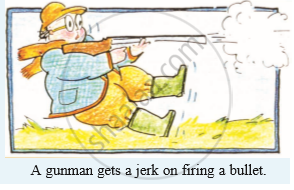Short Answers | Q 19 | Page 74

If action is always equal to reaction, explain why a cart pulled by a horse can be moved.

Short Answers | Q 20 | Page 75

Explain how a rocket works.

Short Answers | Q 21 | Page 75

Do action and reaction act on the same body or different bodies ? How are they related in magnitude and direction ? Are they simultaneous or not ?

Short Answers | Q 22 | Page 75

If a man jumps out from a boat, the boat moves backwards. Why ?

Short Answers | Q 23 | Page 75

Why is it difficult to walk on a slippery road ?

Short Answers | Q 24 | Page 75

Explain why, a runner presses the ground with his feet before he starts his run.

Short Answers | Q 25 | Page 75

A 60 g bullet fired from a 5 kg gun leaves with a speed of 500 m/s. Find the speed (velocity) with which the gun recoils (jerks backwards).

Short Answers | Q 26 | Page 75

A 10 g bullet travelling at 200 m/s strikes and remains embedded in a 2 kg target which is originally at rest but free to move. At what speed does the target move off ?

Short Answers | Q 27 | Page 75

A body of mass 2 kg is at rest. What should be the magnitude of force which will make the body move with a speed of 30 m/s at the end of 1 s ?

Short Answers | Q 28 | Page 75

A body of mass 5 kg is moving with a velocity of 10 m/s. A force is applied to it so that in 25 seconds, it attains a velocity of 35 m/s. Calculate the value of the force applied.

Short Answers | Q 29 | Page 75

A car of mass 2400 kg moving with a velocity of 20 m s-1 is stopped in 10 seconds on applying brakes. Calculate the retardation and the retarding force.

Short Answers | Q 30 | Page 75

For how long should a force of 100 N act on a body of 20 kg so that it acquires a velocity of 100 m/s ?

Short Answers | Q 31 | Page 75

How long will it take a force of 10 N to stop a mass of 2.5 kg which is moving at 20 m/s ?

Short Answers | Q 32 | Page 75

The velocity of a body of mass 10 kg increases from 4 m/s to 8 m/s when a force acts on it for 2 s.
(a) What is the momentum before the force acts ?
(b) What is the momentum after the force acts ?
(c) What is the gain in momentum per second ?
(d) What is the value of the force ?

Short Answers | Q 33 | Page 75

A gun of mass 3 kg fires a bullet of mass 30 g. The bullet takes 0.003 s to move through the barrel of the gun and acquires a velocity of 100 m/s. Calculate :

(i) the velocity with which the gin recoils.
(ii) the force exerted on gunman due to recoil of the gun

Short Answers | Q 34 | Page 75

Draw a diagram to show how a rocket engine provides a force to move the rocket upwards. Label the diagram appropriately.

Short Answers | Q 35.1 | Page 75

Name the law involved in the following situation :

the sum of products of masses and velocities of two moving bodies before and after their collision remains the same.

Short Answers | Q 35.2 | Page 75

Name the law involved in the following situation:

a body of mass 5 kg can be accelerated more easily by a force than another body of mass 50 kg under similar conditions.

Short Answers | Q 35.3 | Page 75

Name the law involved in the following situation :

when person A standing on roller skates pushes another person B (also standing on roller skates) and makes him move to the right side, then the person A himself gets moved to the left side by an equal distance.

Short Answers | Q 35.4 | Page 75

Name the law involved in the following situation :

if there were no friction and no air resistance, then a moving bicycle would go on moving for ever.

Long Answers | Q 36.1 | Page 75

State and explain Newton’s second law of motion.

Long Answers | Q 36.2 | Page 75

A 1000 kg vehicle moving with a speed of 20 m/s is brought to rest in a distance of 50 metres :
(i) Find the acceleration.
(ii) Calculate the unbalanced force acting on the vehicle.

Long Answers | Q 37.1 | Page 75

Explain why, a cricket player moves his hands backwards while catching a fast cricket ball.

Long Answers | Q 37.2 | Page 75

A 150 g ball, travelling at 30 m/s, strikes the palm of a player’s hand and is stopped in 0.05 second. Find the force exerted by the ball on the hand.

Long Answers | Q 38.1 | Page 75

State Newton’s third law of motion and give two examples to illustrate the law.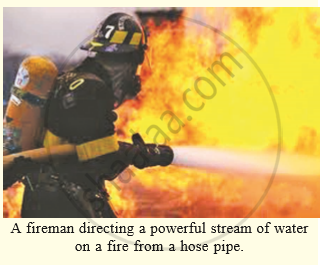Long Answers | Q 38.2 | Page 75

Explain why, when a fireman directs a powerful stream of water on a fire from a hose pipe, the hose pipe tends to go backward.Long Answers | Q 39.1 | Page 76

State the law of conservation of momentum.

Long Answers | Q 39.2 | Page 76

Discuss the conservation of momentum in each of the following cases :
(i) a rocket taking off from ground.
(ii) flying of a jet aeroplane.

Long Answers | Q 40.1 | Page 76

If a balloon filled with air and its mouth untied, is released with its mouth in the downward direction, it
moves upwards. Why ?

Long Answers | Q 40.2 | Page 76

An unloaded truck weighing 2000 kg has a maximum acceleration of 0.5 m/s2. What is the maximum acceleration when it is carrying a load of 2000 kg ?

MCQ | Q 41 | Page 76

The rockets work on the principle of conservation of :

• mass

• energy

• momentum

• velocity

MCQ | Q 42 | Page 76

An object of mass 2 kg is sliding with a constant velocity of 4 m/s on a frictionless horizontal table. The force required to keep this object moving with the same velocity is :

• 32 N

• 0 N

• 2 N

• 8 N

MCQ | Q 43 | Page 76

The physical quantity which makes it easier to accelerate a small car than a large car is measured in the unit of :

• m/s

• kg

• kg.m/s

• kg.m/s2

MCQ | Q 44 | Page 76

According to the third law of motion, action and reaction :

• always act on the same body but in opposite directions

• always act on different bodies in opposite directions

• have same magnitudes and directions

• act on either body at normal to each other

MCQ | Q 45 | Page 76

The unit of measuring momentum of a moving body is :

• m s−1

• kg.ms−1

• kg.m s−2

• Nm2 kg−2

MCQ | Q 46 | Page 76

A boy of mass 50 kg standing on ground exerts a force of 500 N on the ground. The force exerted by the ground on the boy will be :

• 50 N

• 25000 N

• 10 N

• 500 N

MCQ | Q 47 | Page 76

A Honda City car, a Maruti Alto car, A Tata Nano car and a Mahindra Scorpio car, all are running at the same speed of 50 m/s under identical conditions. If all these cars are hit from behind with the same force and they continue to move forward, the maximum acceleration will be produced in :

•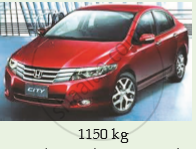•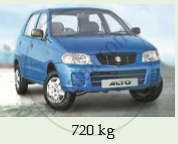•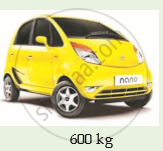•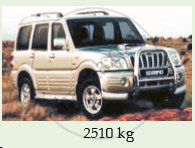MCQ | Q 48 | Page 76

The acceleration produced by a force of 5 N acting on a mass of 20 kg in m/s2 is :

• 4

• 100

• 0.25

• 2.5

MCQ | Q 49 | Page 76

Which of the following situations involves the Newton's second law of motion?

• a force can stop a lighter vehicle as well as a heavier vehicle which are moving

• a force can accelerate a lighter vehicle more easily than a heavier vehicle which are moving

• a force exerted by a lighter vehicle on collision with a heavier vehicle results in both the vehicles coming to a standstill'

• a force exerted by a escaping air from a balloon in the downward direction makes the balloon to go upwards

MCQ | Q 50 | Page 76

A fielder pulls his hands backwards after catching the cricket ball. This enables the fielder to :

•  exert larger force on the ball

• reduce the force exerted by the ball

• increase the rate of change of momentum

•  keep the ball in hands firmly

Hots Questions | Q 51 | Page 77

Why are car seat-belts designed to stretch some what in a collision ?

Hots Questions | Q 52 | Page 77

The troops (soldiers) equipped to be dropped by parachutes from an aircraft are called paratroopers. Why do paratroopers roll on landing ?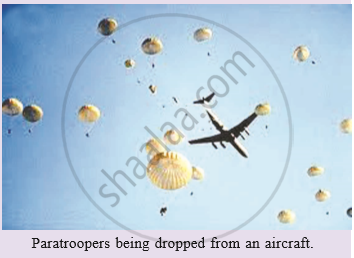Hots Questions | Q 53 | Page 77

Why would an aircraft be unable to fly on the moon ?

Hots Questions | Q 54 | Page 77

Explain why it is possible for a small animal to fall from a considerable height without any injury being caused when it reaches the ground.

Hots Questions | Q 55 | Page 77

A boy of mass 50 kg running at 5 m/s jumps on to a 20 kg trolley travelling in the same direction at 1.5 m/s. What is their common velocity ?

Hots Questions | Q 56 | Page 77

A girl of mass 50 kg jumps out of a rowing boat of mass 300 kg on to the bank, with a horizontal velocity of 3 m/s. With what velocity does the boat begin to move backwards ?

Hots Questions | Q 57 | Page 77

A truck of mass 500 kg moving at 4 m/s collides with another truck of mass 1500 kg moving in the same direction at 2 m/s. What is their common velocity just after the collision if they move off together ?

Hots Questions | Q 58 | Page 77

A ball X of mass 1 kg travelling at 2 m/s has a head-on collision with an identical ball Y at rest. X stops and Y moves off. Calculate the velocity of Y after the collision.

Hots Questions | Q 59 | Page 77

A heavy car A of mass 2000 kg travelling at 10 m/s has a head-on collision with a sports car B of mass 500 kg. If both cars stop dead on colliding, what was the velocity of car B ?

Hots Questions | Q 60 | Page 77

A man wearing a bullet-proof vest stands still on roller skates. The total mass is 80 kg. A bullet of mass 20 grams is fired at 400 m/s. It is stopped by the vest and falls to the ground. What is then the velocity of the man?

Chapter 2: Force and Laws of Motion

Lakhmir Singh Science Class 9 Part 1 PhysicsLakhmir Singh solutions for Class 9 Science chapter 2 - Force and Laws of Motion

Lakhmir Singh solutions for Class 9 Science chapter 2 (Force and Laws of Motion) include all questions with solution and detail explanation. This will clear students doubts about any question and improve application skills while preparing for board exams. The detailed, step-by-step solutions will help you understand the concepts better and clear your confusions, if any. Shaalaa.com has the CBSE Science for Class 9 Part 1 Physics solutions in a manner that help students grasp basic concepts better and faster.

Further, we at Shaalaa.com are providing such solutions so that students can prepare for written exams. Lakhmir Singh textbook solutions can be a core help for self-study and acts as a perfect self-help guidance for students.

Concepts covered in Class 9 Science chapter 2 Force and Laws of Motion are Force and Laws of Motion (Numerical), Conservation of Momentum, Third Law of Motion, Mathematical Formulation of Second Law of Motion, Second Law of Motion, Inertia and Mass, First Law of Motion, Balanced and Unbalanced Forces.

Using Lakhmir Singh Class 9 solutions Force and Laws of Motion exercise by students are an easy way to prepare for the exams, as they involve solutions arranged chapter-wise also page wise. The questions involved in Lakhmir Singh Solutions are important questions that can be asked in the final exam. Maximum students of CBSE Class 9 prefer Lakhmir Singh Textbook Solutions to score more in exam.

Get the free view of chapter 2 Force and Laws of Motion Class 9 extra questions for Science and can use Shaalaa.com to keep it handy for your exam preparation

S